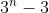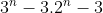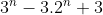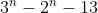# DM - Functions & Relations -Q12

+1 vote

Number of onto functions from a set of n elements (n>2) to set of 3 elements?

(A).(B).(C).(D).asked Jun 24
reshown 6 days ago

+1 vote

This can be done using inclusion and exclusion principles.

Total functions: 3^n

Now, we find the number of functions which are not onto.

Case 1: One element of RHS is not mapped. Every element in LHS will have two choices, hence 2^n, 3 times.

Case 2: Two elements of RHS are not mapped. Every element in LHS will have only one choice. Hence, 1.3

Case 3: Three elements of RHS are not mapped. Zero functions possible.

Hence, answer will be 3^n - 3.2^n + 3answered 4 days ago by (112,390 points)